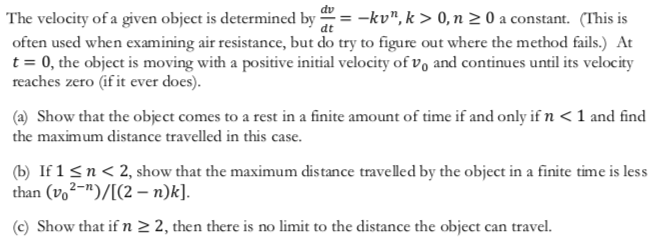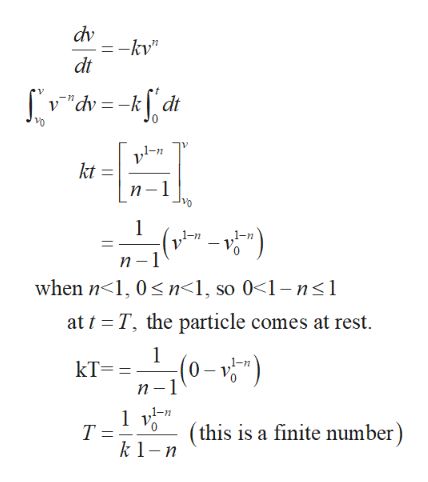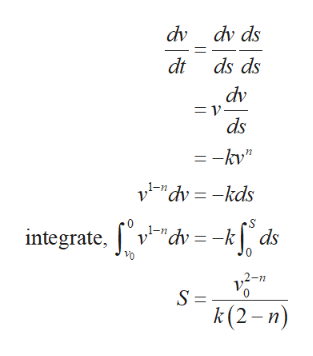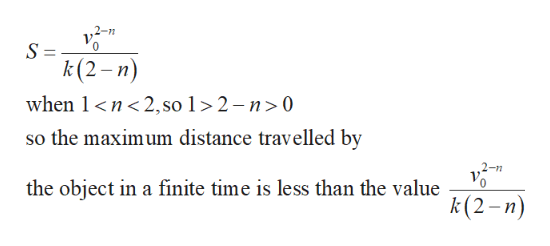# dvThe velocity of a given object is determined byoften used when examining air resistance, but do try to figure out where the method fails.) Att 0, the object is moving with a positive initial velocity of vo and continues until its velocityreaches zero (if it ever does).-kv", k> 0, n 2 0 a constant. (This is(a) Show that the object comes to a rest in a finite amount of time if and only if n < 1 and findthe maximum distance travelled in this case(b If 1 sn

Question
8 viewshelp_outlineImage Transcriptionclosedv The velocity of a given object is determined by often used when examining air resistance, but do try to figure out where the method fails.) At t 0, the object is moving with a positive initial velocity of vo and continues until its velocity reaches zero (if it ever does). -kv", k> 0, n 2 0 a constant. (This is (a) Show that the object comes to a rest in a finite amount of time if and only if n < 1 and find the maximum distance travelled in this case (b If 1 sn<2, show that the maximum distance travelled by the object in a finite time is less than (vo2-)/[(2 n)k]. (c) Show that if n 2 2, then there is no limit to the distance the object can travel. fullscreen
check_circle

Step 1

(a). given that the differential equation describing the velocity of an object.

Integrate the equation,help_outlineImage Transcriptionclosedv =-kv" dt 77 SV"dv=_kj dt kt п -1 - v) 11 п —1 when n 1, 0sn<1, so 0-1-ns1 at t T, the particle comes at rest 1 (0-v") п —1 kT = 1 y-n. Т: k1-n this is a finite number) 0 fullscreen
Step 2

The distance travelled by the object can be calculated as follows,help_outlineImage Transcriptionclosedv dv ds dt ds ds dv = v ds =-kv" vi-"dv-kds 0 integrate,v"dv=-k ds 2-n S = k(2-n) fullscreen
Step 3

(b). the maximum distance travelled by the object in...help_outlineImage TranscriptioncloseS= k(2-n) when 1 n2,so 1 > 2 - n>0 so the maximum distance travelled by the object in a finite time is less than the value k(2-n) fullscreen

### Want to see the full answer?

See Solution

#### Want to see this answer and more?

Solutions are written by subject experts who are available 24/7. Questions are typically answered within 1 hour.*

See Solution
*Response times may vary by subject and question.
Tagged in

### Other